- 5 minutes read

# Performing Calculations on Date- and Time-Related Values

Relational databases support several date and time data types. In this article, we'll look at several arithmetic operations we can do on these types. These operations are logical and understandable, even for the beginning SQL coder.

Let's first briefly explain the main data types used for dates and times. Keep in mind that data types may differ by database engine, so check your database documentation for specifics before you start working with them.

• DATE: Uses three components to represent a specific day: year, month, and day. Dates have many different input formats (i.e. '2016-01-15' or '01/15/16'). Some of them are available in an RDBMS (relational database management system), others are not. Check your documentation for the input format parameters.
• DATETIME: Represents a point in a timeline. A DATETIME value can have different levels of precision. It can store the year, month, day, and hour ('2016-12-19 06'), or even the minutes and seconds ('2016-12-19 06:30:45').
• INTERVAL: Represents elapsed time. Examples of interval values are: "1 day", "2 hours", "2 hours, 30 minutes and 20 seconds", "10 years". An interval is not associated with any specific start time; it is only a duration. Like the DATE data type, an INTERVAL value has different input formats. So you can refer to the same value by using different formats. (Again, these formats depend on the RDBMS you use.)
• TIME: Represents a valid time (i.e. "12:59:59"). It is not frequently used, as TIMESTAMP can represent times.

### An Example Table

We'll use the following SQL code snippet to create an example table:

```CREATE TABLE emergency_dispatch (
emergency_id		integer,
phone_pickup		timestamp,
first_keystroke		timestamp,
unit_assigned		timestamp,
unit_assigned_id	integer,
unit_arrived		timestamp,
help_finished		timestamp
```

Our sample table will have the following data: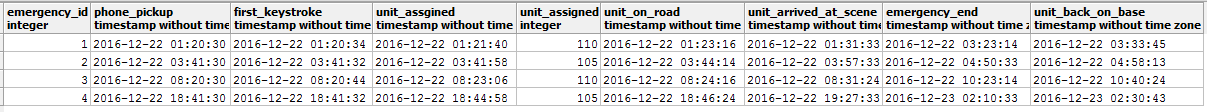Okay. Now let's get started with some basic date-and-time arithmetic.

### Performing Operations with Date and Time Data Types

#### Subtracting Timestamps

As we can see our "`emergency_dispatch`" table has plenty of TIMESTAMP fields. We will cover some interesting operations we can perform on these values.

Suppose we want to have a metric about how much time elapses between answering a call and putting an emergency unit on the road. In this case, we are looking for an interval. How should we calculate it? If we subtract the `unit_on_road` value from the `phone_pickup` value using this operation ...

```SELECT emergency_id, unit_on_road - phone_pickup as time_to_dispatch
FROM emergency_dispatch
```

... We will obtain the elapsed time we are looking for. Notice how the result is stored – in an INTERVAL data type: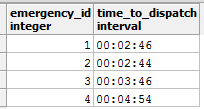The reason behind this operation is that if we subtract two timestamps we will obtain an interval result showing the time that has elapsed between the two values.

Let's go deeper. Suppose we want to obtain the average time needed to react to calls on December 22, 2016 ('2016/12/22'). This is the SQL we'll use:

```SELECT AVG( unit_on_road - phone_pickup)
FROM emergency_dispatch
WHERE phone_pickup::date = '2016/12/22'.
```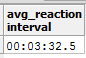#### Adding an INTERVAL and a TIMESTAMP

Let's try another type of calculation. What about adding values from a TIMESTAMP and an INTERVAL? First of all, we are adding two different data types, so what data type can we expect for the result?

If we have a TIMESTAMP "A" and an INTERVAL "B", then adding A and B will produce a TIMESTAMP value representing the instant when B ends, assuming that B started at A.

Let's see an example of how this works:

In our emergency dispatch call center, every operator has two minutes to assign an emergency unit. The following query will detect occasions when the operator exceeded the two-minute threshold:

```Select 	emergency_id,
phone_pickup + CAST('2 minute' AS interval)  as limit_time_to_assign,
unit_assigned
FROM emergency_dispatch
WHERE phone_pickup + CAST('2 minute' AS interval) < unit_assigned
```

The next table shows calls where there was a delay greater than two minutes. Remember, the syntax for the INTERVAL data type may differ from database to database.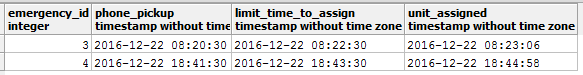With all this knowledge about date and time calculations, we can combine both previous operations to ascertain how much additional time (over two minutes) the call incurred. To do this, we subtract `limit_time_to_assign` from `unit_assigned`. Here's how it's done:

```Select 	emergency_id,
phone_pickup + CAST('2 minute' AS interval) as limit_time_to_assign,
unit_assigned,
unit_assigned - ( phone_pickup+CAST('2 minute' AS interval) ) as 	delay_incurred
FROM emergency_dispatch
WHERE phone_pickup + CAST('2 minute' AS interval) < unit_assigned
```

Below, we can see the `limit_time_to_assign` field and the real time when the unit was assigned. We can also see the new `delay_incurred` interval that was calculated as the difference of the two timestamps.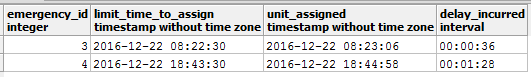There are other calculations we can do with INTERVAL and TIMESTAMP values; in the following table summarizes what is possible and the sort of values returned.

A data type B data type Operation Resulting Data type Meaning
Timestamp Timestamp A – B Interval Elapsed time between B and A
Timestamp Timestamp A + B NOT VALID N/A
Timestamp Interval A + B Timestamp If B begins at A, A+B is the timestamp when B ends
Timestamp Interval A – B Timestamp If B ends at A, A-B is the timestamp when B starts
Interval Interval A + B Interval A longer interval representing the combined duration of A and B
Interval Interval A – B Interval A shorter interval representing duration of A minus duration of B
Interval Integer A * B Interval An interval representing an interval multiplied by a factor of B

### Try It Yourself

There are many different arithmetic operations supported in SQL that involve TIMESTAMP and INTERVAL data types. In the LearnSQL's Standard SQL Functions course, you can learn about many other operations and functions related to date and time calculations. Try it yourself!